# Test: Trees- 1

## 20 Questions MCQ Test GATE Computer Science Engineering(CSE) 2023 Mock Test Series | Test: Trees- 1

Description
Attempt Test: Trees- 1 | 20 questions in 60 minutes | Mock test for Computer Science Engineering (CSE) preparation | Free important questions MCQ to study GATE Computer Science Engineering(CSE) 2023 Mock Test Series for Computer Science Engineering (CSE) Exam | Download free PDF with solutions
QUESTION: 1

### Which of the following is a true about Binary Trees?

Solution:

A full binary tree (sometimes proper binary tree or 2-tree or strictly binary tree) is a tree in which every node other than the leaves has two children. A complete binary tree is a binary tree in which every level, except possibly the last, is completely filled, and all nodes are as far left as possible.

(A) is incorrect. For example, the following Binary tree is neither complete nor full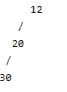(B) is incorrect. The following binary tree is complete but not full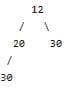(C) is incorrect. Following Binary tree is full, but not complete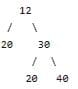(D) is incorrect. Following Binary tree is both complete and full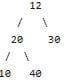QUESTION: 2

### If arity of operators is fixed, then which of the following notations can be used to parse expressions without parentheses? (a) Infix Notation (Inorder traversal of a expression tree) (b) Postfix Notation (Postorder traversal of a expression tree) (c) Prefix Notation (Preorder traversal of a expression tree)

Solution:
• Polish notation (PN), also known as normal Polish notation (NPN), Lukasiewicz notation, Warsaw notation, Polish prefix notation or simply prefix notation, is a mathematical notation in which operators precede their operands, in contrast to reverse Polish notation (RPN) in which operators follow their operands. It does not need any parentheses as long as each operator has a fixed number of operands. The description "Polish" refers to the nationality of logician Jan Lukasiewicz, who invented Polish notation in 1924.
• The term Polish notation is sometimes taken (as the opposite of infix notation) to also include reverse Polish notation.
• When Polish notation is used as a syntax for mathematical expressions by programming language interpreters, it is readily parsed into abstract syntax trees and can, in fact, define a one-to-one representation for the same. Because of this, Lisp (see below) and related programming languages define their entire syntax in terms of prefix notation (and others use postfix notation).
QUESTION: 3

### Level of a node is distance from root to that node. For example, level of root is 1 and levels of left and right children of root is 2. The maximum number of nodes on level i of a binary tree is ____. In the following answers, the operator '^' indicates power.

Solution:

2^(i-1) where i ≧1. Proof: by Induction,
• Introduction base: i=1 (root) The number of node is: 2^(i-1) = 2^0 = 1.
• Induction hypothesis Assume that for i ≧1, the maximum number of nodes on level i-1 is 2^(i-2).
• Induction step Since each node in a binary tree has a maximum degree of 2. Therefore, the maximum number of nodes on level i is 2*2^(i-2) which is 2^(i-1)

QUESTION: 4

In a complete k-ary tree, every internal node has exactly k children or no child. The number of leaves in such a tree with n internal nodes is:

Solution:

For an k-ary tree where each node has k children or no children, following relation holds L = (k-1)*n + 1 Where L is the number of leaf nodes and n is the number of internal nodes. Let us see following for example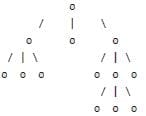k = 3
Number of internal nodes n = 4
Number of leaf nodes = (k-1)*n + 1
= (3-1)*4 + 1
= 9

QUESTION: 5

The maximum number of binary trees that can be formed with three unlabeled nodes is:

Solution: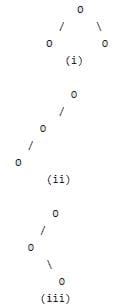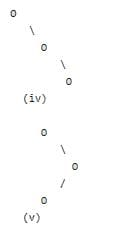Note that nodes are unlabeled. If the nodes are labeled, we get more number of trees.

QUESTION: 6

The number of leaf nodes in a rooted tree of n nodes, with each node having 0 or 3 children is:

Solution:

Let L be the number of leaf nodes and I be the number of internal nodes, then following relation holds for above given tree

L = (3-1)I + 1 = 2I + 1

Total number of nodes(n) is sum of leaf nodes and internal nodes

n = L + I

After solving above two, we get L = (2n+1)/3

QUESTION: 7

A weight-balanced tree is a binary tree in which for each node. The number of nodes in the left sub tree is at least half and at most twice the number of nodes in the right sub tree. The maximum possible height (number of nodes on the path from the root to the farthest leaf) of such a tree on n nodes is best described by which of the following?

Solution:

Let the maximum possible height of a tree with n nodes is represented by H(n). The maximum possible value of H(n) can be approximately written using following recursion

H(n) = H(2n/3) + 1

The solution of above recurrence is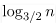. We can simply get it by drawing a recursion tree.

QUESTION: 8

A complete n-ary tree is a tree in which each node has n children or no children. Let I be the number of internal nodes and L be the number of leaves in a complete n-ary tree. If L = 41, and I = 10, what is the value of n?

Solution:

For an n-ary tree where each node has n children or no children, following relation holds

L = (n-1)*I + 1

Where L is the number of leaf nodes and I is the number of internal nodes. Let us find out the value of n for the given data.

L = 41 , I = 10
41 = 10*(n-1) + 1
(n-1) = 4
n = 5

QUESTION: 9

The height of a binary tree is the maximum number of edges in any root to leaf path. The maximum number of nodes in a binary tree of height h is:

Solution:

Maximum number of nodes will be there for a complete tree.
Number of nodes in a complete tree of height h = 1 + 2 + 22 + 2*3 + …. 2h = 2(h+1) – 1

QUESTION: 10

A scheme for storing binary trees in an array X is as follows. Indexing of X starts at 1 instead of 0. the root is stored at X. For a node stored at X[i], the left child, if any, is stored in X[2i] and the right child, if any, in X[2i+1]. To be able to store any binary tree on n vertices the minimum size of X should be.

Solution:

For a right skewed binary tree, number of nodes will be 2n - 1. For example, in below binary tree, node 'A' will be stored at index 1, 'B' at index 3, 'C' at index 7 and 'D' at index 15.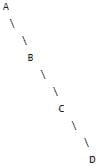QUESTION: 11

Postorder traversal of a given binary search tree, T produces the following sequence of keys 10, 9, 23, 22, 27, 25, 15, 50, 95, 60, 40, 29 Which one of the following sequences of keys can be the result of an in-order traversal of the tree T?

Solution:

Inorder traversal of a BST always gives elements in increasing order. Among all four options, a) is the only increasing order sequence.

QUESTION: 12

Consider the following nested representation of binary trees: (X Y Z) indicates Y and Z are the left and right sub stress, respectively, of node X. Note that Y and Z may be NULL, or further nested. Which of the following represents a valid binary tree?

Solution:

(1 (2 3 4)(5 6 7)) represents following binary tree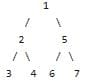(A) (1 2 (4 5 6 7)) is not fine as there are 4 elements in one bracket.

(B) (1 (2 3 4) 5 6) 7) is not fine as there are 2 opening brackets and 3 closing.

(D) (1 (2 3 NULL) (4 5)) is not fine one bracket has only two entries (4 5)

QUESTION: 13

Consider a node X in a Binary Tree. Given that X has two children, let Y be Inorder successor of X. Which of the following is true about Y?

Solution:

Since X has both children, Y must be leftmost node in right child of X.

QUESTION: 14

In a binary tree with n nodes, every node has an odd number of descendants. Every node is considered to be its own descendant. What is the number of nodes in the tree that have exactly one child?

Solution:

It is mentioned that each node has odd number of descendants including node itself, so all nodes must have even number of descendants 0, 2, 4 so on. Which means each node should have either 0 or 2 children. So there will be no node with 1 child. Hence 0 is answer. Following are few examples.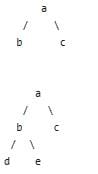Such a binary tree is full binary tree (a binary tree where every node has 0 or 2 children).

QUESTION: 15

The inorder and preorder traversal of a binary tree are d b e a f c g and a b d e c f g, respectively. The postorder traversal of the binary tree is:

Solution:

Below is the given tree.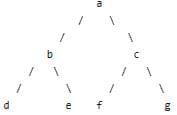QUESTION: 16

The height of a tree is the length of the longest root-to-leaf path in it. The maximum and minimum number of nodes in a binary tree of height 5 are:

Solution:

Number of nodes is maximum for a perfect binary tree. A perfect binary tree of height h has 2h+1 - 1 nodes.
Number of nodes is minimum for a skewed binary tree. A perfect binary tree of height h has h+1 nodes.

QUESTION: 17

A binary tree T has 20 leaves. The number of nodes in T having two children is

Solution:

Sum of all degrees = 2 * |E|. Here considering tree as a k-ary tree :

Sum of degrees of leaves + Sum of degrees for Internal Node except root + Root's degree = 2 * (No. of nodes - 1). Putting values of above terms,
L + (I-1)*(k+1) + k = 2 * (L + I - 1)
L + k*I - k + I -1 + k = 2*L + 2I - 2
L + K*I + I - 1 = 2*L + 2*I - 2
K*I + 1 - I = L
(K-1)*I + 1 = L
Given k = 2, L=20
⇒ (2-1)*I + 1 = 20
⇒ I = 19
⇒ T has 19 internal nodes which are having two children.

QUESTION: 18

Consider a complete binary tree where the left and the right subtrees of the root are max-heaps. The lower bound for the number of operations to convert the tree to a heap is

Solution:

The answer to this question is simply max-heapify function. Time complexity of max-heapify is O(Log n) as it recurses at most through height of heap.

// A recursive method to heapify a subtree with root at given index
// This method assumes that the subtrees are already heapified void MinHeap::MaxHeapify(int i)

{
int l = left(i);
int r = right(i);
int largest = i;
if (l < heap_size && harr[l] < harr[i]) largest = l;
if (r < heap_size && harr[r] < harr[smallest]) largest = r;
if (largest != i) { swap(&harr[i], &harr[largest]); MinHeapify(largest);
}
}

QUESTION: 19

An array of integers of size n can be converted into a heap by adjusting the heaps rooted at each internal node of the complete binary tree starting at the node ⌊(n – 1) /2⌋, and doing this adjustment up to the root node (root node is at index 0) in the order ⌊(n – 1)/2⌋, ⌊(n – 3)/ 2⌋, ….., 0. The time required to construct a heap in this manner is:

Solution:

BUILD-HEAP(A)

heapsize := size(A);

for i := floor(heapsize/2) downto 1

do HEAPIFY(A, i);

end for

END

Upper bound of time complexity is O(n) for above algo

QUESTION: 20

What are the main applications of tree data structure?
1) Manipulate hierarchical data.
2) Make information easy to search (see tree traversal).
3) Manipulate sorted lists of data.
4) Router algorithms.
5) Form of a multi-stage decision-making, like Chess Game.
6) As a workflow for compositing digital images for visual effects

Solution:Use Code STAYHOME200 and get INR 200 additional OFF Use Coupon Code